• 主要介绍了Python对列表排序的方法,实例分析了Python列表排序函数的相关使用技巧,非常简单实用,需要的朋友可以参考下
• 使用Python内置sort()方法用来排序，也可以用python内置的全局sorted()方法来对可迭代的序列排序生成新的序列。 sorted()方法： 简单的升序排序是非常容易的。只需要调用sorted()方法。它返回一个新的list，新的...
基础排序
使用Python内置sort()方法用来排序，也可以用python内置的全局sorted()方法来对可迭代的序列排序生成新的序列。

sorted()方法
简单的升序排序是非常容易的。只需要调用sorted()方法。它返回一个新的list，新的list的元素基于小于运算符(__lt__)来排序。
sorted()语法
sorted(iterable, key=None, reverse=False)  #注在python2中该函数还有个cmp参数，不过在3中取消了

参数：

iterable -- 可迭代对象。
cmp(了解)：用于比较的函数，比较什么由key决定;
key -- 主要是用来进行比较的元素，只有一个参数。用列表元素的某个属性或函数作为关键字，有默认值，迭代集合中的一项(指定可迭代对象中的一个元素来进行排序。)
reverse -- 排序规则，reverse = True 降序 ， reverse = False 升序（默认）。

返回值：

返回重新排序的列表。
参数说明： (1)  cmp参数(了解)：cmp接受一个函数，拿整形举例，形式为:
def f(a,b):
return a-b
如果排序的元素是其他类型的，如果a逻辑小于b，函数返回负数(-1)；a逻辑等于b，函数返回0；a逻辑大于b，函数返回正数(1)就行了   (2)  key参数：key也是接受一个函数，不同的是，这个函数只接受一个元素,形式如下
def f(a):
return len(a)
key接受的函数返回值，表示此元素的权值，sorted将按照权值大小进行排序   (3) reverse参数：接受False 或者True 表示是否逆序

简单排序
例1:
list = [5, 2, 3, 1, 4]

new_list = sorted(list)

print(new_list,list)

#[1, 2, 3, 4, 5] [5, 2, 3, 1, 4]
例1_1:
list = [5, 2, 3, 1, 4]

new_list = sorted(list,reverse = True)

print(new_list,list)

#[5, 4, 3, 2, 1] [5, 2, 3, 1, 4]

关键字排序
例2：
list = [('b',2),('a',1),('c',3),('d',4)]

new_list = sorted(list, key=lambda x:x,reverse=True) #序列为第一个参数的序列(x表示按任意元素中索引为1的元素排序)

print(new_list)

#[('d', 4), ('c', 3), ('b', 2), ('a', 1)]
例3：
List = [{1:5,3:4},{1:3,6:3},{1:1,2:4,5:6},{1:9}]

def f(x):
return len(x)

new_list = sorted(List,key = f)    #按照元素长度排序

print(new_list)

#[{1: 9}, {1: 5, 3: 4}, {1: 3, 6: 3}, {1: 1, 2: 4, 5: 6}]
例4：
List = ['Chr1-10.txt','Chr1-1.txt','Chr1-2.txt',
'Chr1-14.txt',
'Chr1-3.txt','Chr1-20.txt','Chr1-5.txt']

def func(element):
return int(element.split('-').split('.'))
#对元素进行连续两次切片操作(比较函数的参数表示列表中的任意元素)

new_list = sorted(List, key=func)
#new_list = sorted(List, key=lambda d : int(d.split('-')[-1].split('.')))

print(new_list)

#['Chr1-1.txt', 'Chr1-2.txt', 'Chr1-3.txt', 'Chr1-5.txt', 'Chr1-10.txt', 'Chr1-14.txt', 'Chr1-20.txt']
注： 这就是key的功能，制定排序的关键字，通常都是一个lambda函数，当然你也可以事先定义好这个函数。如果不将这个关键字转化为整型，结果是这样的：
sorted(s, key=lambda d : d.split('-')[-1].split('.'))

['Chr1-1.txt', 'Chr1-10.txt', 'Chr1-14.txt', 'Chr1-2.txt', 'Chr1-20.txt', 'Chr1-3.txt', 'Chr1-5.txt']
这相当于把这个关键字当做字符串了，很显然，在python中，'2' > '10'
你可以定制你想要的key, 如 key = lambda x : len(x) 按照序列的长度去排序。key= lambda x : (x, x) 按二个元素，再第一个 等等。。。
例5：
from operator import itemgetter, attrgetter

students = [('john', 'A', 15),
('jane', 'B', 12),
('dave', 'B', 10),]

new_student = sorted(students, key=lambda student : student)   # sort by age(索引为2的元素排序)

new_student_1 = sorted(students, key=itemgetter(2))#根据第二个域进行排序

new_student_2 = sorted(students, key=itemgetter(1,2))  # sorted by grade then by age(有相同值时，根据多个元素排序)

print(new_student)
print(new_student_1)
print(new_student_2)

"""
[('dave', 'B', 10), ('jane', 'B', 12), ('john', 'A', 15)]
[('dave', 'B', 10), ('jane', 'B', 12), ('john', 'A', 15)]
[('john', 'A', 15), ('dave', 'B', 10), ('jane', 'B', 12)]
"""

对由字典排序
例6：
from operator import itemgetter, attrgetter

d = {'data1':3, 'data2':1, 'data3':2, 'data4':4}

new_d = sorted(d.items(), key=itemgetter(1), reverse=True)
#d.items():使用items()先将字典转换为二元组的列表

print(new_d)

#[('data4', 4), ('data1', 3), ('data3', 2), ('data2', 1)]
注：返回的是由tuple组成的List，不再是字典。

对字符串进行排序
例7：
str = "This is a test string from Andrew"

split_str = str.split(" ")
print("原序列:",split_str)

new_str = sorted(split_str,key=lambda x:x)

new_str_1 = sorted(split_str,key=lambda x: x.lower())

print("区分大小写的排序：",new_str)
print("不区分大小写的排序：",new_str_1)

"""
原序列: ['This', 'is', 'a', 'test', 'string', 'from', 'Andrew']
区分大小写的排序： ['Andrew', 'This', 'a', 'from', 'is', 'string', 'test']
不区分大小写的排序： ['a', 'Andrew', 'from', 'is', 'string', 'test', 'This']
"""

sort()函数
描述 sort()函数用于对原列表进行排序，如果指定参数，则使用比较函数指定的比较函数。语法
sort()方法语法：

list.sort(cmp=None, key=None, reverse=False)

参数
cmp(了解) -- 可选参数, 如果指定了该参数会使用该参数的方法进行排序。
key -- 主要是用来进行比较的元素，只有一个参数，具体的函数的参数就是取自于可迭代对象中，指定可迭代对象中的一个元素来进行排序。
reverse -- 排序规则，reverse = True 降序， reverse = False 升序（默认）。

返回值
该方法没有返回值，但是会对列表的对象进行排序。
例8：
aList = ['Google', 'Runoob', 'Taobao', 'Facebook']

aList.sort()
print("List : ", aList)

#List :  ['Facebook', 'Google', 'Runoob', 'Taobao']
例9：
# 获取列表的第二个元素
def takeSecond(elem):
return elem

# 列表
random = [(2, 2), (3, 4), (4, 1), (1, 3)]
random_1 = [(2, 2), (3, 4), (4, 1), (1, 3)]

# 指定第二个元素排序
random.sort( key= takeSecond)
random_1.sort(key=lambda x:x)

# 输出类别
print('排序列表：', random)
print('排序列表：', random_1)

"""
排序列表： [(4, 1), (2, 2), (1, 3), (3, 4)]
排序列表： [(1, 3), (2, 2), (3, 4), (4, 1)]
"""
注：sort 与 sorted 区别：
1、sort ()是应用在 list 上的方法，sorted 可以对所有可迭代的对象进行排序操作。
2、list 的 sort 方法返回的是对已经存在的列表进行操作，无返回值，而内建函数 sorted 方法返回的是一个新的 list，而不是在原来的基础上进行的操作。

备注
在python2.4前，sorted()和list.sort()函数没有提供key参数，但是提供了cmp参数来让用户指定比较函数。此方法在其他语言中也普遍存在。
在python3.0中，cmp参数被彻底的移除了，从而简化和统一语言，减少了高级比较和__cmp__方法的冲突。
在python2.x中cmp参数指定的函数用来进行元素间的比较。此函数需要2个参数，然后返回负数表示小于，0表示等于，正数表示大于。例如：
>>> def numeric_compare(x, y):
return x - y
>>> sorted([5, 2, 4, 1, 3], cmp=numeric_compare)
[1, 2, 3, 4, 5]
当我们将现有的2.x的代码移植到3.x时，需要将cmp函数转化为key函数：使用functools模块中的cmp_to_key()函数例10：
from functools import cmp_to_key

list = [{1:5,3:4},{1:3,6:3},{1:1,2:4,5:6},{1:9}]

def f2(a,b):
return a-b

new_list = sorted(list,key = cmp_to_key(f2))
#按照每个字典元素里面key为1的元素的值排序

print(new_list)

#[{1: 1, 2: 4, 5: 6}, {1: 3, 6: 3}, {1: 5, 3: 4}, {1: 9}]


展开全文• ## python对列表排序

千次阅读 2018-10-11 16:10:37
1、基础排序：sorted list=sorted([5, 2, 3, 1, 4]) print(list) 输出结果: [1, 2, 3, 4, 5] 2.key参数/函数 key参数的值为一个函数，此函数只有一个参数且返回一个值用来进行比较。 例如通过key指定的函数来...
1、基础排序：sorted
list=sorted([5, 2, 3, 1, 4])
print(list)
输出结果:
[1, 2, 3, 4, 5]

2.key参数/函数
key参数的值为一个函数，此函数只有一个参数且返回一个值用来进行比较。
例如通过key指定的函数来忽略字符串的大小写：
list=sorted("This is a test string from Andrew".split(), key=str.lower)
打印结果：
['a', 'Andrew', 'from', 'is', 'string', 'test', 'This']
但是key参数更多的是用复杂对象的某些值来对复杂对象的序列排序：
复杂语法：
sorted(iterable, cmp=None, key=None, reverse=False) ===》
iterable：是可迭代类型; cmp：用于比较的函数，比较什么由key决定; key：用列表元素的某个属性或函数进行作为关键字，有默认值，迭代集合中的一项; reverse：排序规则. reverse = True  降序 或者 reverse = False 升序，有默认值。 返回值：是一个经过排序的可迭代类型，与iterable一样。
举例：lambda：
sorted_list=sorted(four_list,key=lambda x:x["hit_count"],reverse=True)
解释：four_list：要排序的列表；lambda是一个匿名函数，主体是个表达式，根据“hit_count”排序；reverse=True代表降序
最简单的lambda例子：定义一个lambda表达式，求三个数的和
# -*- coding: UTF-8 -*-
f = lambda x,y,z:x + y + z

print f(1,2,3)
print f(4,5,6)

输出：
6
15


展开全文• 列表排序 方法1: 没有返回值 list1 = [2, 1, 4, 5, 3] print("最开始数据:{}".format(list1)) # 升序 list1.sort() print("升序后数据:{}".format(list1)) list1 = [2, 1, 4, 5, 3] print("最开始数据:{}"....
一  列表的排序
方法1: 没有返回值
list1 = [2, 1, 4, 5, 3]
print("最开始数据:{}".format(list1))
# 升序
list1.sort()
print("升序后数据:{}".format(list1))
list1 = [2, 1, 4, 5, 3]
print("最开始数据:{}".format(list1))
# 降序
list1.sort(reverse=True)
print("升序后数据:{}".format(list1))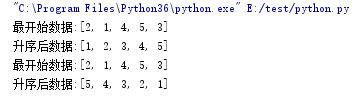2.方法2:有返回值
list1 = [2, 1, 4, 5, 3]
print("最开始数据:{}".format(list1))
# 升序
list2 = sorted(list1)
print("升序后数据:{}".format(list2))
list1 = [2, 1, 4, 5, 3]
print("最开始数据:{}".format(list1))
# 降序
list2 = sorted(list1, reverse=True)
print("升序后数据:{}".format(list2))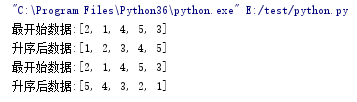二 字典的排序
方式1:
里面三个参数
dict1.items() #可迭代元素。
key= lambda dict1:dict1  #dict1表示按键，dict1表示按值。
reverse #默认是False，升序排列。当值为True时是降序排列。
按照键进行排序
dict1 = {"name": "Kevin", "age": "27", "gender": "true"}
# 升序
dict2 = sorted(dict1.items(), key=lambda dict1: dict1, reverse=False)
print("开始的字典数据:{}".format(dict1))
print("按照键进行升序后的数据:{}".format(dict2))

dict1 = {"name": "Kevin", "age": "27", "gender": "true"}
# 降序
dict2 = sorted(dict1.items(), key=lambda dict1: dict1, reverse=True)
print("开始的字典数据:{}".format(dict1))
print("按照键进行降序后的数据:{}".format(dict2))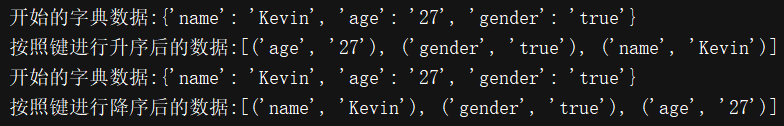按照值进行排序
dict1 = {"name": "Kevin", "age": "27", "gender": "true"}
# 升序
dict2 = sorted(dict1.items(), key=lambda dict1: dict1, reverse=False)
print("开始的字典数据:{}".format(dict1))
print("按照值进行升序后的数据:{}".format(dict2))

dict1 = {"name": "Kevin", "age": "27", "gender": "true"}
# 降序
dict2 = sorted(dict1.items(), key=lambda dict1: dict1, reverse=True)
print("开始的字典数据:{}".format(dict1))
print("按照值进行降序后的数据:{}".format(dict2))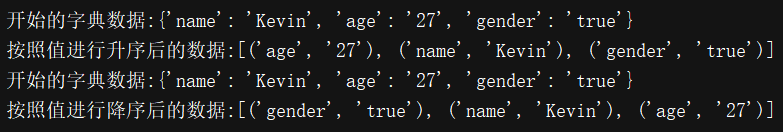三 包含字典dict的列表list的排序方法
方法1：使用 operator进行排序
import operator

list1 = [{'name': 'Kevin', 'age': 27}, {'name': 'Ling', 'age': 23}]
# 升序
list2 = sorted(list1, key=operator.itemgetter('age'))
print("开始列表:{}".format(list1))
print("升序后列表:{}".format(list2))

# 降序
list2 = sorted(list1, key=operator.itemgetter('age'), reverse=True)
print("开始列表:{}".format(list1))
print("降序后列表:{}".format(list2))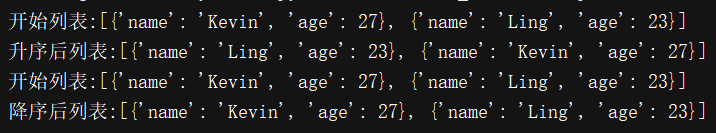方法1：使用 lambda 进行排序

list1 = [{'name': 'Kevin', 'age': 27}, {'name': 'Ling', 'age': 23}]
# 升序
list2 = sorted(list1, key=lambda list1: list1["age"])
print("开始列表:{}".format(list1))
print("升序后列表:{}".format(list2))

# 降序
list2 = sorted(list1, key=lambda list1: list1["age"], reverse=True)
print("开始列表:{}".format(list1))
print("降序后列表:{}".format(list2))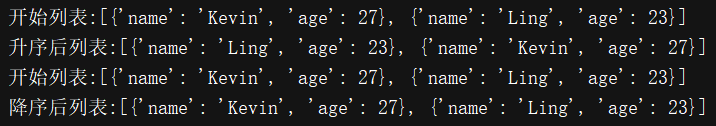展开全文字典
• 关于Python列表排序 sort排序方法 ​ 这个方法可以对列表元素进行排列（默认正向排序），排序后生成的新列表会对原列表进行覆盖（但其id不变），所以sort排序方法会直接修改原列表的排序。 a =[1,3,787,33,86,99] a...
• ## python-列表排序

千次阅读 2019-02-27 20:52:50
输入三个整数x, y, z，形成一个列表，请把这n个数由小到大输出 这就是要求升序排列，用之前写过几种方法 法一 #数组 li=[1,3,5,7,2,0] # 用sort()排序 li_sort = sorted(li, reverse = False) print('用...
输入三个整数x, y, z，形成一个列表，请把这n个数由小到大输出
这就是要求升序排列，用之前写过几种方法
法一
#数组
li=[1,3,5,7,2,0]

# 用sort()排序
li_sort = sorted(li, reverse = False)
print('用sort方法，排列结果：{}'.format(li_sort))


法二：冒泡排序
# 冒泡排序
def bubbleSort(m):
m = m.copy()
for time in range(1, len(m)):
for index in range(len(m) - time):
if m[index] > m[index+1]:
m[index], m[index+1] = m[index+1] , m[index]
return  m

print(bubbleSort(li))

法三：选择排序

# 选择排序
def selectSort(m):
m = m.copy()
for seat_L in range(len(m)-1):
for seat_R in range(seat_L+1, len(m)):
if m[seat_L] > m[seat_R]:
m[seat_L], m[seat_R] = m[seat_R], m[seat_L]
return m

print(selectSort(li))


法三：插入排序
def insertSort(m):
m = m.copy()
result = m[:1]
for index_choose in range(1, len(m)):
for index_insert in range(len(result) + 1):
print(result, index_insert,'\n',m, index_choose,'\n\n')
if index_insert != index_choose and m[index_choose] < result[index_insert]:
result.insert(index_insert, m[index_choose])
break
if index_insert == index_choose:
result.append(m[index_choose])
return result
print(insertSort(li))

法四：希尔排序
    import math
def shellSort(arr):
gap=1
while(gap < len(arr)/3):
gap = gap*3+1
while gap > 0:
for i in range(gap,len(arr)):
temp = arr[i]
j = i-gap
while j >=0 and arr[j] > temp:
arr[j+gap]=arr[j]
j-=gap
arr[j+gap] = temp
gap = math.floor(gap/3)
return arr


展开全文• ## Python 的列表排序

万次阅读 2011-12-16 10:05:15
Horin | horin153@msn.com  Blog: ...  在 Python 中, 当需要对一个 list 排序时, 一般可以用 list.sort() 或者 sorted(iterable[, cmp[, key[, reverse]]]). 其中: cmp(e1, e2) 是带lambda import list pair
• ## python列表排序

千次阅读 2013-07-17 11:19:08
python列表排序 简单记一下python中List的sort方法（或者sorted内建函数）的用法。 关键字： python列表排序 python字典排序 sorted List的元素可以是各种东西，字符串，字典，自己定义的类等。 sorted函数用法...
• 今天，我们一起看一下python中关于列表排序的一些知识。 有些时候我们希望对列表进行排序后，列表可以保存我们排序后的结果，但是很多情况下我们只是希望通过列表的排序，临时的显示排序结果而已。所以对于列表的...
• 以下总结了python列表排序，基本上覆盖了所有问题。 # -*- coding: utf-8 -*- import sys reload(sys) sys.setdefaultencoding('UTF-8') '''列表排序''' '''数据需要排序时，往往会把数据格式转化为列表进行排序'...
• 本文大家介绍的是Python列表排序方法中的reverse、sort、sorted操作方法，以及他们直接的区别介绍，有需要的小伙伴可以参考下。
• 主要介绍了Python实现的列表排序、反转操作,结合实例形式分析了Python针对列表的sort排序、以及基于reverse、切片的反转操作相关实现技巧,需要的朋友可以参考下
• 很多时候，我们需要对List进行排序Python提供了两个方法，对给定的List L进行排序： 方法1.用List的成员函数sort进行排序 方法2.用built-in函数sorted进行排序（从2.4开始） 这两种方法使用起来差不多，以第一种为...
• 列表嵌套怎么进行排序呢？ 噔噔噔！！用lambda就可以很简洁的进行排序啦！下面介绍一下具体步骤： 这是一份班级学生的成绩信息集合：字段包括学号，姓名，语文成绩，数学成绩 item=[[101,'张三',80,75],[102,'...
• 在本篇文章里小编大家分享了关于python实现列表排序方法以及相关知识点，有兴趣的朋友们可以学习下。
• ## Python列表的排序

千次阅读 2017-01-20 15:54:57sort zip
• 【题目】python中的排序函数sorted以及列表排序方法sort（)   概述 Python list内置sort()方法用来排序，也可以用python内置的全局sorted()方法来对可迭代的序列排序生成新的序列。如果要读取文件夹下面的文件，...sorted() reverse
• 嵌套列表排序 列表： demolist = [[3,'b','2020-7-1'],[2,'e','2020-6-26'],[10,'a','2020-8-16']] 排序： demolist.sort(key=lambda x: x, reverse=False) 说明： reverse为False则按从大到小降序排列，为True...
• 参考：Equivalent of Numpy.argsort() in basic python? - Stack Overflow 通过 enumerate 实现 [i for i,v in sorted(enumerate(['Vincent', 'Alex', 'Bill', 'Matthew']), key=lambda x:x)] output: [1...
• 手记-- encoding=utf-8 --python3代码import operator一. 按字典值排序（默认为升序）x = {1:2, 3:4, 4:3, 2:1, 0:0}sorted_x = sorted(x.iteritems(), key=operator.itemgetter(1))print sorted_x[(0, 0), (2, 1), ...
• ## PTA Python列表排序

千次阅读 2018-12-18 23:49:57
输入由10个整数构成的列表，编程只对列表中下标为偶数的元素进行升续排序，下标为奇数的元素保持不动。 输入格式: 输入列表。 输出格式: 输出排序后的列表。 输入样例: 在这里出一组输入。例如： 52,49,80,36,14,...
• Python中字典的排序分为按“键”排序和按“值”排序。 1、按“值”排序  按“值”排序就是根据字典的值进行排序，可以使用内置的sorted()函数。  sorted(iterable[, cmp[, key[, reverse]]]...

# python给列表排序python 订阅